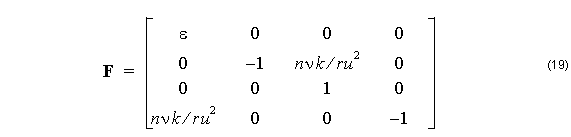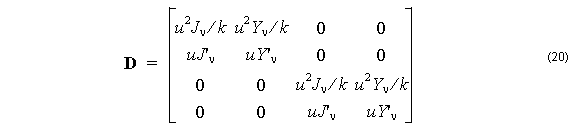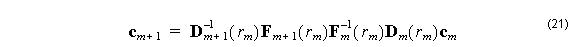The order of the components in the vector f is chosen to help factor the matrix in (9) into two matrices as Ff = Dc where c = [ A, B, C, D ]T  ,The advantage of this factoring is that it is easy to find the expressions for F–1  and D–1 .

F–1  is found by two row reductions and D–1  by inverting the 2×2 matrices in the block diagonal. These matrices are required to find the coefficients in the next layer. If  cm are the coefficients in the m-th layer, then the coefficients in the next layer is found from the matrix product,The use of the factored matrix means four matrix multiplications instead of two are required to cross the boundary, but it avoids having to find the matrix inverse numerically.